# Newton's laws of motion 14 april 2015

19 Apr 2015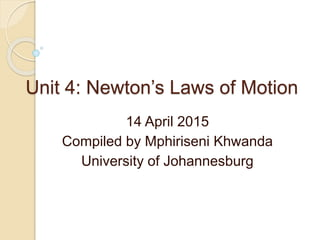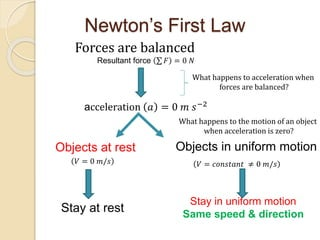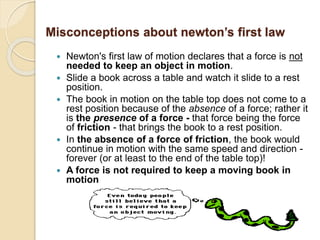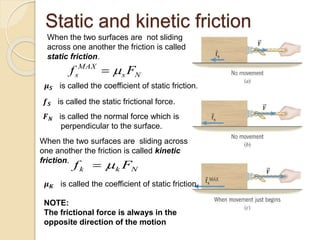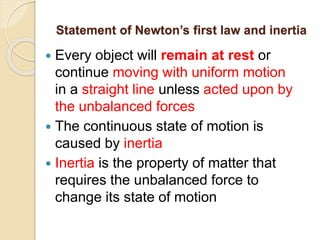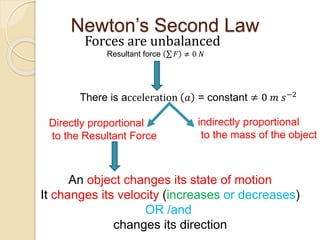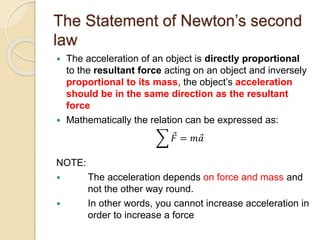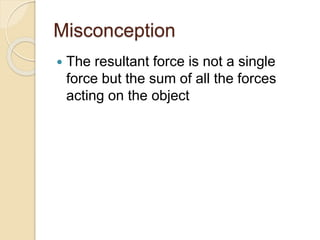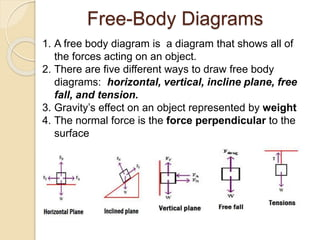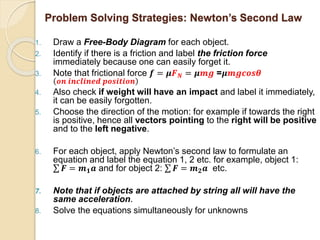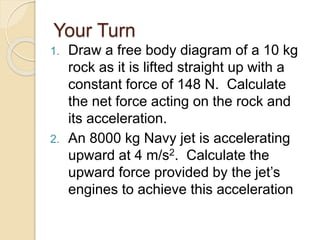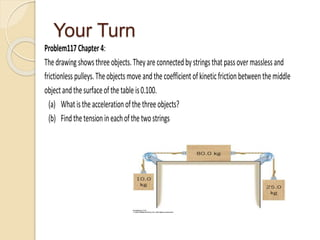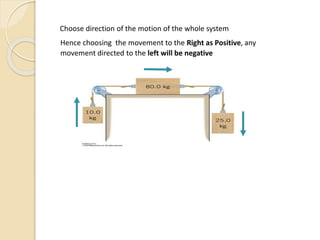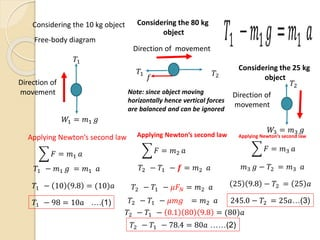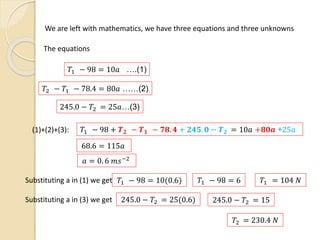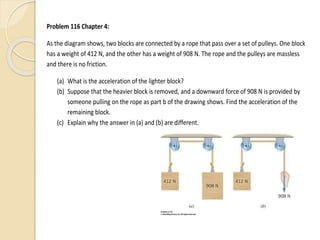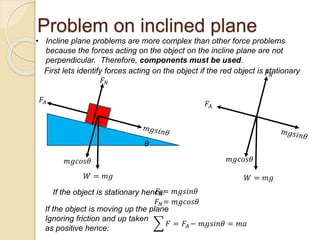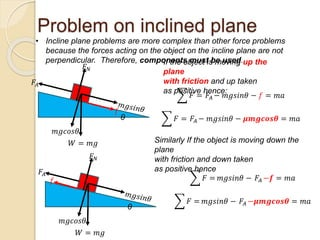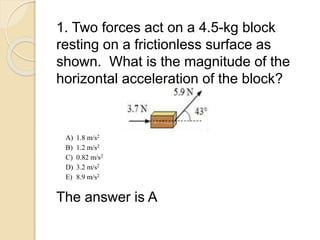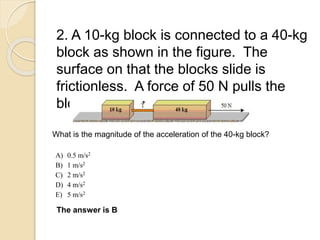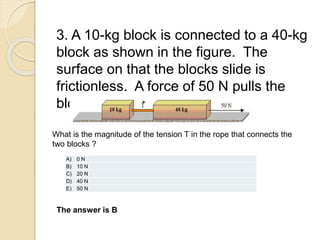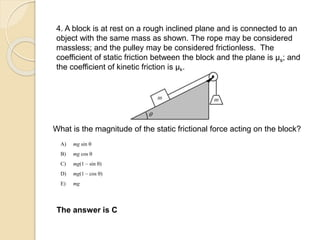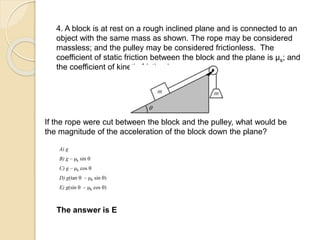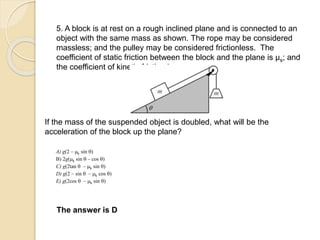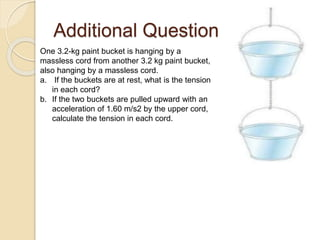1 sur 25

### Newton's laws of motion 14 april 2015

• 1. Unit 4: Newton’s Laws of Motion 14 April 2015 Compiled by Mphiriseni Khwanda University of Johannesburg
• 2. Newton’s First Law Forces are balanced Resultant force 𝐹 = 0 𝑁 acceleration 𝑎 = 0 𝑚 𝑠−2 Objects at rest Objects in uniform motion Stay at rest Stay in uniform motion Same speed & direction 𝑉 = 0 𝑚/𝑠 𝑉 = 𝑐𝑜𝑛𝑠𝑡𝑎𝑛𝑡 ≠ 0 𝑚/𝑠 What happens to acceleration when forces are balanced? What happens to the motion of an object when acceleration is zero?
• 3. Misconceptions about newton’s first law  Newton's first law of motion declares that a force is not needed to keep an object in motion.  Slide a book across a table and watch it slide to a rest position.  The book in motion on the table top does not come to a rest position because of the absence of a force; rather it is the presence of a force - that force being the force of friction - that brings the book to a rest position.  In the absence of a force of friction, the book would continue in motion with the same speed and direction - forever (or at least to the end of the table top)!  A force is not required to keep a moving book in motion
• 4. Static and kinetic friction When the two surfaces are not sliding across one another the friction is called static friction. 𝝁 𝑺 is called the coefficient of static friction. 𝒇 𝑺 is called the static frictional force. 𝑭 𝑵 is called the normal force which is perpendicular to the surface. Ns MAX s Ff  When the two surfaces are sliding across one another the friction is called kinetic friction. 𝝁 𝑲 is called the coefficient of static friction. Nkk Ff  NOTE: The frictional force is always in the opposite direction of the motion
• 5. Statement of Newton’s first law and inertia  Every object will remain at rest or continue moving with uniform motion in a straight line unless acted upon by the unbalanced forces  The continuous state of motion is caused by inertia  Inertia is the property of matter that requires the unbalanced force to change its state of motion
• 6. Newton’s Second Law Forces are unbalanced Resultant force 𝐹 ≠ 0 𝑁 There is acceleration 𝑎 = constant ≠ 0 𝑚 𝑠−2 indirectly proportional to the mass of the object An object changes its state of motion It changes its velocity (increases or decreases) OR /and changes its direction Directly proportional to the Resultant Force
• 7. The Statement of Newton’s second law  The acceleration of an object is directly proportional to the resultant force acting on an object and inversely proportional to its mass, the object’s acceleration should be in the same direction as the resultant force  Mathematically the relation can be expressed as: 𝐹 = 𝑚 𝑎 NOTE:  The acceleration depends on force and mass and not the other way round.  In other words, you cannot increase acceleration in order to increase a force
• 8. Misconception  The resultant force is not a single force but the sum of all the forces acting on the object
• 9. Free-Body Diagrams 1. A free body diagram is a diagram that shows all of the forces acting on an object. 2. There are five different ways to draw free body diagrams: horizontal, vertical, incline plane, free fall, and tension. 3. Gravity’s effect on an object represented by weight 4. The normal force is the force perpendicular to the surface
• 10. Problem Solving Strategies: Newton’s Second Law 1. Draw a Free-Body Diagram for each object. 2. Identify if there is a friction and label the friction force immediately because one can easily forget it. 3. Note that frictional force 𝒇 = 𝝁𝑭 𝑵 = 𝝁𝒎𝒈 =𝝁𝒎𝒈𝒄𝒐𝒔𝜽 𝒐𝒏 𝒊𝒏𝒄𝒍𝒊𝒏𝒆𝒅 𝒑𝒐𝒔𝒊𝒕𝒊𝒐𝒏 4. Also check if weight will have an impact and label it immediately, it can be easily forgotten. 5. Choose the direction of the motion: for example if towards the right is positive, hence all vectors pointing to the right will be positive and to the left negative. 6. For each object, apply Newton’s second law to formulate an equation and label the equation 1, 2 etc. for example, object 1: 𝑭 = 𝒎 𝟏 𝒂 and for object 2: 𝑭 = 𝒎 𝟐 𝒂 etc. 7. Note that if objects are attached by string all will have the same acceleration. 8. Solve the equations simultaneously for unknowns
• 11. Your Turn 1. Draw a free body diagram of a 10 kg rock as it is lifted straight up with a constant force of 148 N. Calculate the net force acting on the rock and its acceleration. 2. An 8000 kg Navy jet is accelerating upward at 4 m/s2. Calculate the upward force provided by the jet’s engines to achieve this acceleration
• 13. Choose direction of the motion of the whole system Hence choosing the movement to the Right as Positive, any movement directed to the left will be negative
• 14. Considering the 10 kg object Free-body diagram Direction of movement 𝑇1 − 𝑚1 𝑔 = 𝑚1 𝑎 𝑇1 𝑊1 = 𝑚1 𝑔 Applying Newton’s second law 𝐹 = 𝑚1 𝑎 𝑇1 − 10 9.8 = (10)𝑎 𝑇1 − 98 = 10𝑎 ….(1) Considering the 80 kg object 𝑇1 𝑓 𝑇2 Direction of movement Note: since object moving horizontally hence vertical forces are balanced and can be ignored Applying Newton’s second law 𝐹 = 𝑚2 𝑎 𝑇2 − 𝑇1 − 𝒇 = 𝑚2 𝑎 𝑇2 − 𝑇1 − 𝜇𝐹 𝑁 = 𝑚2 𝑎 𝑇2 − 𝑇1 − 𝜇𝑚𝑔 = 𝑚2 𝑎 𝑇2 − 𝑇1 − 0.1 80 9.8 = (80)𝑎 𝑇2 − 𝑇1 − 78.4 = 80𝑎 ……(2) Considering the 25 kg object 𝑊3 = 𝑚3 𝑔 𝑇2 Direction of movement Applying Newton’s second law 𝐹 = 𝑚3 𝑎 𝑚3 𝑔 − 𝑇2 = 𝑚3 𝑎 25 9.8 − 𝑇2 = 25 𝑎 245.0 − 𝑇2 = 25𝑎…(3)
• 15. We are left with mathematics, we have three equations and three unknowns The equations 𝑇1 − 98 = 10𝑎 ….(1) 𝑇2 − 𝑇1 − 78.4 = 80𝑎 ……(2) 245.0 − 𝑇2 = 25𝑎…(3) (1)+(2)+(3): 𝑇1 − 98 + 𝑻 𝟐 − 𝑻 𝟏 − 𝟕𝟖. 𝟒 + 𝟐𝟒𝟓. 𝟎 − 𝑻 𝟐 = 10𝑎 +𝟖𝟎𝒂 +25𝑎 68.6 = 115𝑎 𝑎 = 0. 6 𝑚𝑠−2 Substituting a in (1) we get 𝑇1 − 98 = 10(0.6) 𝑇1 − 98 = 6 𝑇1 = 104 𝑁 Substituting a in (3) we get 245.0 − 𝑇2 = 25(0.6) 245.0 − 𝑇2 = 15 𝑇2 = 230.4 𝑁
• 16. Problem 116 Chapter 4: As the diagram shows, two blocks are connected by a rope that pass over a set of pulleys. One block has a weight of 412 N, and the other has a weight of 908 N. The rope and the pulleys are massless and there is no friction. (a) What is the acceleration of the lighter block? (b) Suppose that the heavier block is removed, and a downward force of 908 N is provided by someone pulling on the rope as part b of the drawing shows. Find the acceleration of the remaining block. (c) Explain why the answer in (a) and (b) are different.
• 17. Problem on inclined plane • Incline plane problems are more complex than other force problems because the forces acting on the object on the incline plane are not perpendicular. Therefore, components must be used. θ 𝑊 = 𝑚𝑔 𝑚𝑔𝑐𝑜𝑠𝜃 𝐹𝐴 𝐹 𝑁 First lets identify forces acting on the object if the red object is stationary 𝑊 = 𝑚𝑔 𝑚𝑔𝑐𝑜𝑠𝜃 𝐹 𝑁 𝐹𝐴 If the object is stationary hence:𝐹𝐴= 𝑚𝑔𝑠𝑖𝑛𝜃 𝐹 𝑁= 𝑚𝑔𝑐𝑜𝑠𝜃 If the object is moving up the plane Ignoring friction and up taken as positive hence: 𝐹 = 𝐹𝐴 − 𝑚𝑔𝑠𝑖𝑛𝜃 = 𝑚𝑎
• 18. Problem on inclined plane • Incline plane problems are more complex than other force problems because the forces acting on the object on the incline plane are not perpendicular. Therefore, components must be used. θ 𝑚𝑔𝑐𝑜𝑠𝜃 𝐹𝐴 𝐹 𝑁 𝑊 = 𝑚𝑔 If the object is moving up the plane with friction and up taken as positive hence: 𝐹 = 𝐹𝐴 − 𝑚𝑔𝑠𝑖𝑛𝜃 − 𝑓 = 𝑚𝑎 𝐹 = 𝐹𝐴 − 𝑚𝑔𝑠𝑖𝑛𝜃 − 𝝁𝒎𝒈𝒄𝒐𝒔𝜽 = 𝑚𝑎 Similarly If the object is moving down the plane with friction and down taken as positive hence 𝐹 = 𝑚𝑔𝑠𝑖𝑛𝜃 − 𝐹𝐴 −𝒇 = 𝑚𝑎 𝐹 = 𝑚𝑔𝑠𝑖𝑛𝜃 − 𝐹𝐴 −𝝁𝒎𝒈𝒄𝒐𝒔𝜽 = 𝑚𝑎 𝑓 θ 𝑚𝑔𝑐𝑜𝑠𝜃 𝐹𝐴 𝐹 𝑁 𝑊 = 𝑚𝑔 𝑓
• 19. 1. Two forces act on a 4.5-kg block resting on a frictionless surface as shown. What is the magnitude of the horizontal acceleration of the block? The answer is A A) 1.8 m/s2 B) 1.2 m/s2 C) 0.82 m/s2 D) 3.2 m/s2 E) 8.9 m/s2
• 20. 2. A 10-kg block is connected to a 40-kg block as shown in the figure. The surface on that the blocks slide is frictionless. A force of 50 N pulls the blocks to the right. What is the magnitude of the acceleration of the 40-kg block? A) 0.5 m/s2 B) 1 m/s2 C) 2 m/s2 D) 4 m/s2 E) 5 m/s2 The answer is B
• 21. 3. A 10-kg block is connected to a 40-kg block as shown in the figure. The surface on that the blocks slide is frictionless. A force of 50 N pulls the blocks to the right. What is the magnitude of the tension T in the rope that connects the two blocks ? The answer is B A) 0 N B) 10 N C) 20 N D) 40 N E) 50 N
• 22. 4. A block is at rest on a rough inclined plane and is connected to an object with the same mass as shown. The rope may be considered massless; and the pulley may be considered frictionless. The coefficient of static friction between the block and the plane is µs; and the coefficient of kinetic friction is µk. What is the magnitude of the static frictional force acting on the block? A) mg sin q B) mg cos q C) mg(1 – sin q) D) mg(1 – cos q) E) mg The answer is C
• 23. 4. A block is at rest on a rough inclined plane and is connected to an object with the same mass as shown. The rope may be considered massless; and the pulley may be considered frictionless. The coefficient of static friction between the block and the plane is µs; and the coefficient of kinetic friction is µk. If the rope were cut between the block and the pulley, what would be the magnitude of the acceleration of the block down the plane? The answer is E A) g B) g – k sin q C) g – k cos q D) g(tan q – k sin q) E) g(sin q – k cos q)
• 24. 5. A block is at rest on a rough inclined plane and is connected to an object with the same mass as shown. The rope may be considered massless; and the pulley may be considered frictionless. The coefficient of static friction between the block and the plane is µs; and the coefficient of kinetic friction is µk. If the mass of the suspended object is doubled, what will be the acceleration of the block up the plane? The answer is D A) g(2 – k sin q) B) 2g(k sin q – cos q) C) g(2tan q – k sin q) D) g(2 – sin q – k cos q) E) g(2cos q – k sin q)
• 25. Additional Question One 3.2-kg paint bucket is hanging by a massless cord from another 3.2 kg paint bucket, also hanging by a massless cord. a. If the buckets are at rest, what is the tension in each cord? b. If the two buckets are pulled upward with an acceleration of 1.60 m/s2 by the upper cord, calculate the tension in each cord.